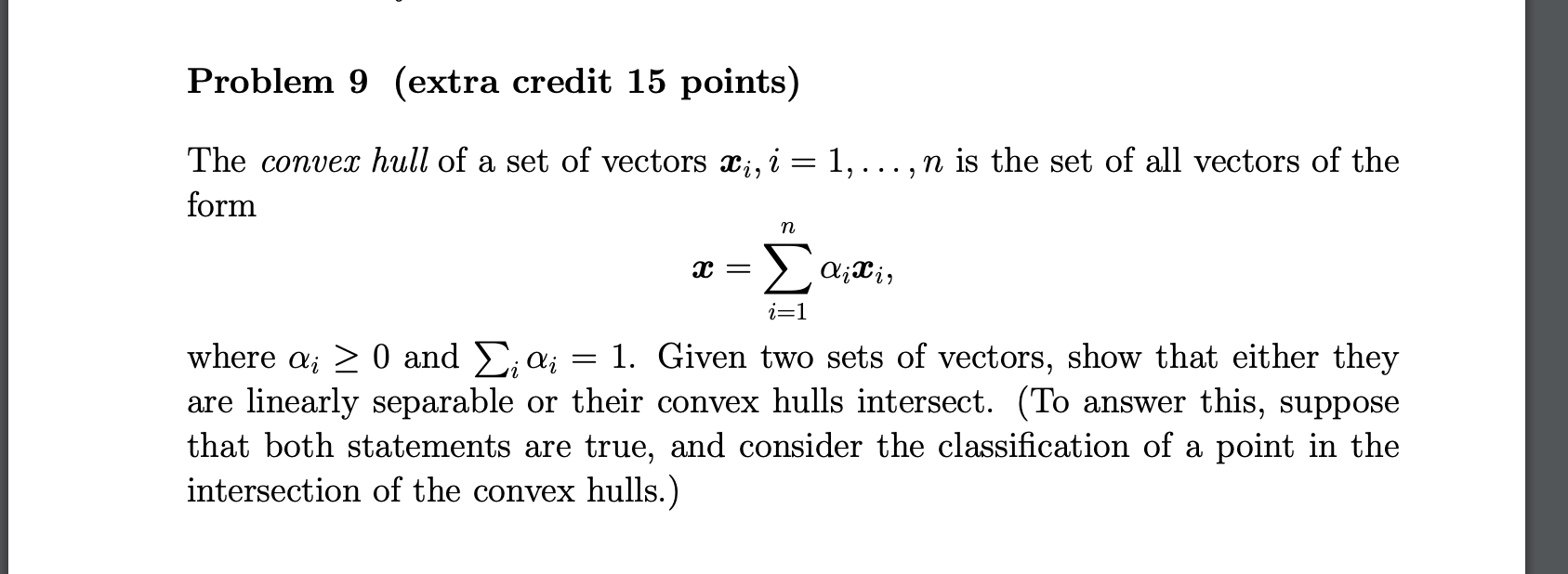# Question Solved1 Answer9) The convex hull of a set of vectors xi , i = 1, . . . , n is the set of all vectors of the form x = X n i=1 αixi , where αi ≥ 0 and P i αi = 1. Given two sets of vectors, show that either they are linearly separable or their The convex $$h u l l$$ of a set of vectors $$\boldsymbol{x}_{i}, i=1, \ldots, n$$ is the set of all vectors of the form $\boldsymbol{x}=\sum_{i=1}^{n} \alpha_{i} \boldsymbol{x}_{i}$ where $$\alpha_{i} \geq 0$$ and $$\sum_{i} \alpha_{i}=1$$. Given two sets of vectors, show that either they are linearly separable or their convex hulls intersect. (To answer this, suppose that both statements are true, and consider the classification of a point in the intersection of the convex hulls.)9) The convex hull of a set of vectors xi , i = 1, . . . , n is the set of all vectors of the form x = X n i=1 αixi , where αi ≥ 0 and P i αi = 1. Given two sets of vectors, show that either they are linearly separable or their convex hulls intersect. (To answer this, suppose that both statements are true, and consider the classification of a point in the intersection of the convex hulls.)

Transcribed Image Text: The convex $$h u l l$$ of a set of vectors $$\boldsymbol{x}_{i}, i=1, \ldots, n$$ is the set of all vectors of the form $\boldsymbol{x}=\sum_{i=1}^{n} \alpha_{i} \boldsymbol{x}_{i}$ where $$\alpha_{i} \geq 0$$ and $$\sum_{i} \alpha_{i}=1$$. Given two sets of vectors, show that either they are linearly separable or their convex hulls intersect. (To answer this, suppose that both statements are true, and consider the classification of a point in the intersection of the convex hulls.)
More
Transcribed Image Text: The convex $$h u l l$$ of a set of vectors $$\boldsymbol{x}_{i}, i=1, \ldots, n$$ is the set of all vectors of the form $\boldsymbol{x}=\sum_{i=1}^{n} \alpha_{i} \boldsymbol{x}_{i}$ where $$\alpha_{i} \geq 0$$ and $$\sum_{i} \alpha_{i}=1$$. Given two sets of vectors, show that either they are linearly separable or their convex hulls intersect. (To answer this, suppose that both statements are true, and consider the classification of a point in the intersection of the convex hulls.)